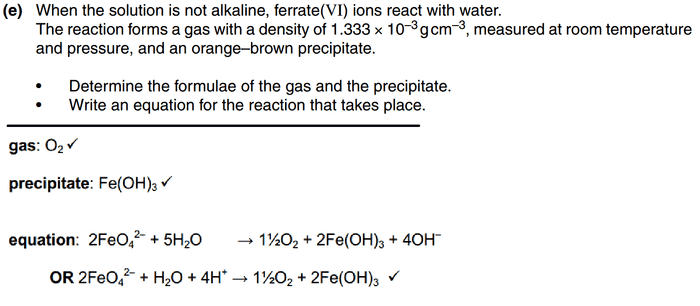# A2 chemistry - how do i know there's an OH in the products?

This is the question and the answer, the problem is i don't know how to get to the answer
top is question and bottom is answerI attempted to divide the gas concentration by 24 to get molar mass, but that didn't work
and how am i suppose to know that OH- is one of the products? i guessed that there is fe(OH)3, but that's all i got right....

it's the very last question from this A level paper

Borek
Mentor
I attempted to divide the gas concentration by 24 to get molar mass

I see no concentration in the question.

And why do you divide? You have mass of 1 mL, right? Assuming there is one mole of a gas at RTP, what volume does it occupy? What is its mass (given the density as listed)?

It is not that there is OH- between products, however, you won't be able to balance the reaction without either H+ of OH-. That's a quite common situation when it comes to redox reactions in water.

epenguin
Homework Helper
Gold Member
1.3333 × 22 = very close to 32 so that part works well.